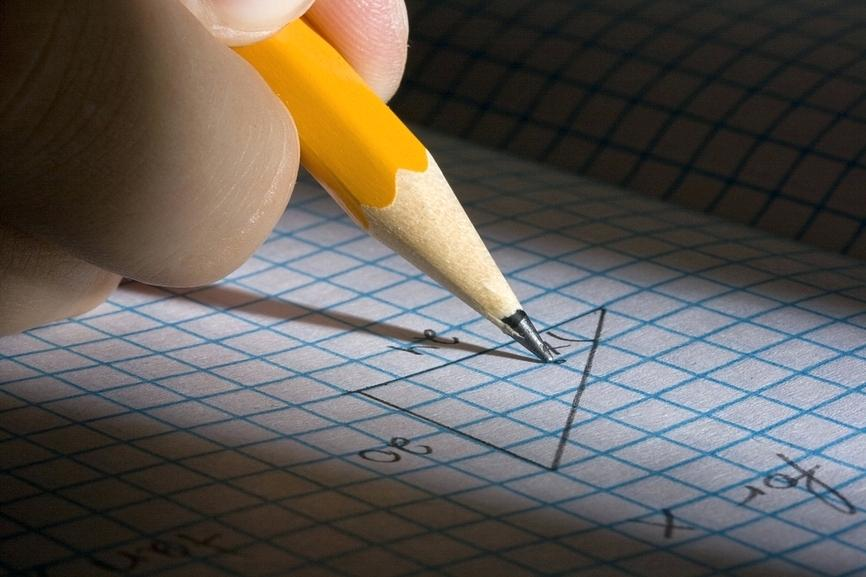Friday, December 1# Easy Way to Learn Geometry Formulas

0
21Mathematics is an integral part of learning for all children. Introducing Maths to children from an early age helps to develop their problem-solving skills, reasoning skills and logical thinking in a broad range of contexts. Numeracy and shapes improve spatial awareness. It helps every child to recognise, create and describe patterns, which is necessary for the early problem-solving skills.

Geometry is a part of a student’s curriculum from kindergarten to higher secondary education. It also continues through college and throughout the entire lifetime. Even without opening the geometry book, it is used daily by almost everyone. It explores geometric reasoning and spatial sense. It is involved in all the disciplines such as Engineering, Space, Art and Architecture, Astronomy, Robotics etc.

Geometry is the central concept that links some topics in Maths, especially in measurement. In schooling, the main focus of geometry is learning about the mensuration formulas. But children find difficulties in learning those formulas. Here, the article provides some easy ways to learn geometry formulas.

## Understand the Concept

To learn the geometry formulas, first, understand the concepts and parameters of different shapes. Clear with concepts such as two-dimensional and three-dimensional geometry. The parameters such as length, breadth, height, altitude, slant height, area, volume, etc. are used in geometry.

## Learn the Relationship Between Different Shapes

Each shape has different characteristics. But some properties of geometrical shape relates to the other form. If you know the relationship between the shapes, it is easy to learn the formulas on your own. If you are learning 3D shape formulas, try to relate with the respective 2D shape. Because 3D shapes are obtained from the rotation of 2D shapes.

## Practice

Once you understand the formulas, write it down and practice it. Practising the formulas, again‌ ‌and‌ ‌again, makes you so familiar with that. For many shapes, the pi value defines the property of that shape. For example, the area of a circle is πr2. If you remove π, it does not represent the circle area.

Subscribe to BYJU’S YouTube Channel to learn the formulas for different geometrical shapes and also explore videos to learn with ease.